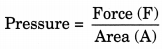On this page, you will find Force and Pressure Class 8 Notes Science Chapter 11 Pdf free download. CBSE NCERT Class 8 Science Notes Chapter 11 Force and Pressure will seemingly help them to revise the important concepts in less time.

## CBSE Class 8 Science Notes Chapter 11 Force and Pressure

### Force and Pressure Class 8 Notes Understanding the Lesson

1. A push or pull on an object is called force.

2. Forces applied on an object in the same direction add to one another.

3. If two forces act in the opposite directions on an object, the net force acting on it is the difference between the two forces.

4. The strength of a force is usually expressed by its magnitude.

5. Force on an object may change its state of motion, speed, direction and shape.

6. The force resulting due to the action of muscles is known as the muscular force.

7. Muscular force can only be applied when it is in contact with an object, it is also called as contact force.

8. The force responsible for changing the state of motion of an object is known as the force of friction.

9. The force exerted on an object without touching it is known as non-contact force.

10. The force exerted by a charged body on another charged or uncharged body is known as electrostatic force.

11. The attractive force of the earth which acts upon all the objects is known as the force of gravity or just gravity. This force is known as gravitational force.

12. The force acting on a unit area of a surface is called pressure.

13. The envelop of air around us is known as atmosphere.

14. The pressure exerted by the atmosphere is known as atmospheric pressure.

### Class 8 Science Chapter 11 Notes Important Terms

Atmospheric pressure: The pressure exerted by air around us is termed as atmospheric pressure.

Contact force: The type of force which acts only when the object are in physical contact with each other is called contact force.

Electrostatic force: The force exerted by an electrified body is called electrostatic force.

Force: A push or pull which tends to change the state of rest, motion direction, size and shape is called as force.

Friction: The force acting between two surfaces in contact which opposes the motion of one body over the another body is called the force of friction.

Gravitational force: The force of attraction between any two objects which have mass is called gravitational force.

Gravity: The force of gravitation which acts on the bodies close to the surface of the earth is called the force of gravity of the earth.

Magnetic force: The type of force which is exerted by a magnet is called magnetic force.

Muscular force: The type of force which is exerted by the muscles of a human or animal body is called muscular force.

Non-contact force: The type of force which is exerted by a body on another body without any direct contact with it is called non-contact force.

Pressure: Force acting per unit area is called pressure.Pull: The type of force which move the object towards source of the force.

Push: The type of force which move the object forward or away from the source of force.